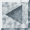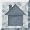Dealing with Noisy Data in the Rough Set MethodTypes of Noise

The most destructive kind of noise in the rough set method is the case where two objects are identical in all condition attributes (C) but different in the decision attribute (D). This disqualifies both objects from being in the positive region and makes k(C, D) < 1.

Consider the information system presented in the following table:

 Condition Attributes Decision Attributes
 Name Hair Height Weight Lotion Result Sarah blonde average light no sunburned (positive) Dana blonde tall average yes none (negative) Alex brown short average yes none Annie blonde short average no sunburned Emily red average heavy no sunburned George red average heavy no none Pete brown tall heavy no none John brown average heavy no none Katie blonde short light yes none

In this case, Emily and George are indiscernible, but one got a sunburn and the other did not. Thus k(C, D) = 7/9 = 78%.

the objects of the universe are people (Sarah, Dana, Alex, Annie, Emily, Pete, John, Katie). Suppose we wish to describe the set X of people who did get a sunburn (X = {Sarah, Annie, Emily}). When using the set of attributes B = C = {Hair, Height, Weight, Lotion}, we find that each equivalence class has exactly one element, so we can define X precisely as B*(X) = X = B*(X) = [Sarah] ∪ [Annie] ∪ [Emily]. If, however, we consider only the attribute Lotion, then there are only two equivalence classes:
• [Sarah] = {Sarah, Annie, Emily, Pete, John}
• [Dana] = {Dana, Alex, Katie}
Observe that we now cannot define X precisely, because [Sarah] contains X. We can, however, make the observation that [Dana] is disjoint from X, so we have the following:
∅ = B*(X) ⊂ X ⊂ B*(X) = [Sarah] ⊂ U.
In terms of rules, the above indicates that not wearing lotion may (but not necessarily) lead to sunburn, and that wearing lotion prevents sunburn.

Reduct of Attributes

In order to simplify the learned rules, we will be interested in eliminating condition attributes. First, we will look for absolute reducts, which are subsets B of the set C of condition attributes such that B preserves the indiscernibility equivalence classes of C. In the "sunburn" example above, we observe that taking B = {Height, Weight, Lotion} (i.e. eliminating Hair) leaves every element of the universe distinct. Other absolute reducts include the following:

• {Hair, Height, Lotion}
• {Hair, Height, Weight}

Consider, however, that for our purposes, absolute reducts are too strict. That is, we do not care if we make formerly discernible objects to become indiscernible, as long as they have the same value for the decision attribute(s). For example, eliminating the attribute Height makes Pete and John indiscernible, but since neither of them got a sunburn, we have not lost any ability to predict a sunburn. Thus, B = {Hair, Weight, Lotion} is a relative reduct of C with respect to D. Notice that Height is in every absolute reduct, but it need not be in a relative reduct. Next, we will discuss these concepts formally.

Positive Regions and Dependency

• Let S = (U, A, V) be an information system, with A = C ∪ D and B ⊂ C.
• Let IND(B) denote the set of equivalence classes of U with respect to B.
• Let IND(D) denote the set of equivalence classes of U with respect to D.

Define the positive region of B in IND(D) as

POSB(D) = ∪{ B*(X) : XIND(D) }.

That is, POSB(D) includes all objects that can be sorted into classes of IND(D) without error, based solely on the classification information in IND(B).

Furthermore, we say that the set of attributes D depends in degree k on the subset R of C if

k(R, D) = card(POSR(D)) ÷ card(POSC(D))

Clearly, k(R, D)K(C, D). Also, the following are equivalent:

• k(R, D) = k(C, D).
• R is a reduct of C with respect to D.
• POSR(D) = POSC(D).
Also, since we have a numerical description of dependency, we can speak of near-perfect reducts (i.e. k(R, D)k(C, D)), so that we may discard even more attributes if they have little effect on the dependency.

Computing the Best Reduct

In general, the problem of finding all reducts is NP-hard. Therefore, we will consider a greedy algorithm for finding the "best" reduct.

Now, it is known that there is a (possibly empty) subset of attributes, called the core, which is common to all reducts. Furthermore, it can be shown that CO = CORE(C, D) = {a ∈ C : POSC(D) ≠ POSC-{a}(D)}. So, we test every attribute to see if it belongs to the core, then we pass the core to the following algorithm:

1. REDU = CO.
2. AR = C - REDU.
3. While k(REDU, D) < k(C, D) - margin, do the following:
1. Find attribute a ∈ AR such that k(REDU ∪ {a}, D) is maximized.
2. Set REDU = REDU ∪ {a}; AR = AR - {a}.
4. Output REDU.
This algorithm is greedy because it hopes to minimize the number of attributes by choosing the attribute that creates the greatest increase in k at each step.

Example

Consider the "sunburn" data above. First, let us compute the core. Every object is discernible, so POSC(D) = U.

Attribute (a)IND(C - {a})POSC - {a}(D)In Core?
Hair{Sarah}, {Dana}, {Alex}, {Annie}, {Emily, John}, {Pete}, {Katie}U - {Emily, John}Yes
Height{Sarah}, {Dana}, {Alex}, {Annie}, {Emily}, {Pete, John}, {Katie}UNo
Weight{Sarah}, {Dana}, {Alex}, {Annie}, {Emily}, {Pete}, {John}, {Katie}UNo
Lotion{Sarah}, {Dana}, {Alex}, {Annie}, {Emily}, {Pete}, {John}, {Katie}UNo

So, CORE(C, D) = CO = {Hair}, and POSCO(D) = {Alex, Emily, Pete, John}, and k(CO, D) = 4/8 = 0.5. Now we proceed with the algorithm.

StepREDUARa ∈ ARk(REDU ∪ {a}, D)Choice
1{Hair}{Height, Weight, Lotion} Height0.5Lotion
Weight0.5
Lotion1.0

The algorithm terminates after one iteration, with the answer REDU = {Hair, Lotion}. The reduced table is as follows:

C D Vote Hair 2 Blonde No Yes 2 Blonde Yes No 1 Brown Yes No 2 Brown No No 1 Red No Yes

Learning Rules

Before generating rules, we want to combine similar tuples. Tuples are similar if they differ in only one attribute (call it a). Ideally, the similar tuples, taken together, would have all possible values for a (known as saturation), but this is not necessary. In the example, notice that Brown/Yes/No and Brown/No/No differ only in the Lotion attribute, and that Lotion is saturated. Therefore, we can combine these into one tuple (Brown/?/No with vote = 3). Similarly, if we assume that Blonde, Brown, and Red are the only hair colours, then we can combine Blonde/No/Yes with Red/No/Yes to get ¬Brown/No/Yes. So the simplified table is as follows:

C D Vote Hair 3 ¬Brown No Yes 2 Blonde Yes No 3 Brown ? No

So, finally, we are ready to read the rules out of the table:

• (Hair ≠ Brown ∧ Lotion = No) ⇒ Sunburn = Yes
• (Hair = Blonde ∧ Lotion = Yes) ∨ (Hair = Brown) ⇒ Sunburn = No